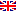Scheda programma d'esame
STATISTICS
CATERINA GIUSTI
Code549PP
Credits12
PeriodSemester 2
LanguageEnglish

ModulesAreaTypeHoursTeacher(s)
STATISTICS ISECS-S/01LEZIONI84
 CATERINA GIUSTI unimap AGNESE MARCELLI unimap
STATISTICS IISECS-S/01LEZIONI0
 00000 000000 unimapEsporta in pdf
Programma non disponibile nella lingua selezionata
Learning outcomes
Knowledge

The aim of the course is introducing students to statistical literacy, i.e. the ability to correctly understand and use data and statistical information with special reference to business and economics data.

Students are expected to acquire knowledge of descriptive statistics such as central values (mean, geometric mean, mode, quantiles), measures of variability (variance, standard deviation), graphical representations (barplot, pie chart, scatterplot, boxplot); some knowledge of probability and a focus on the most common discrete and continuous random variables (discrete uniform, binomial, poisson, hypergeometric, uniform, normal, exponential); basic knowledge of the statistical inference, with a special focus on interval estimation and test of hypothesis for the mean of one normal population and for one population proportion; interval estimation and test of hypothesis about means and proportions with two populations; basic knowledge and inferences for the ANOVA and for the simple and multiple linear regression model.

Assessment criteria of knowledge

In a written exam, the student must demonstrate his/her knowledge of the course material and to be able to solve exercises of descriptive and inferential statistics.

Skills

On successful completion of this course, students will acquire interpretation abilities and critical skills for evaluating statistical information, and the capability to produce and analyse statistical data.

Assessment criteria of skills

During the final exam, the ability to apply the basic statistical methods learned during the course will be evaluated.

Behaviors

Students will be able to develop sensitivity to statistical reasoning and issues related to the use of statistical data (production, analysis and interpretation), with a special reference to business data.

Assessment criteria of behaviors

Part of the final exam is designed to verify the sensitivity to statistical reasoning and general issues related to the use of statistical data.

Prerequisites

Logic and basic mathematics.

Teaching methods

Face to face lectures, with visual aids such as powerpoints presentations.

The teaching materials will be downloadable from the elearning webpage of the course.

Syllabus

The course provides an introduction to the basic methods and tools for the quantitative study of collective phenomena. During the course the following topics will be discussed: Introduction to reasoning and statistical language. Descriptive statistics: tables and graphs; central tendency and dispersion; association between categorical variables, correlation and linear regression. Inferential statistics: probability theory; sampling distributions; estimation and confidence intervals; hypothesis testing (one sample and two samples).

Bibliography

Reference textbook:

Anderson/Sweeney/Williams/Camm/Cochran "Essentials of Statistics for Business and Economics". Cengage, 8th edition. ISBN:9781337114172

Note: older editions of the book can be used.

Assessment methods

The exam consists of a written exam containing exercises to be solved, and questions on the theory and related discussion. The exam is not passed if the written evidence is insufficient.